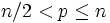# Symmetric group on finite set has Sylow subgroup of prime order

Suppose$n$ is a natural number greater than$1$. Let$S_n$ be the Symmetric group (?) of degree$n$. Then, there exists a prime$p$ such that$S_n$ has a$p$-Sylow subgroup (?) of order$p$, i.e., it is a Group of prime order (?).
1. Bertrand's postulate: The version we use states that for any$n \ge 2$, there exists a prime$p$ such that$p \le n < 2p$.
The symmetric group of degree$n$ has order$n!$. By fact (1), there exists a prime$p$ such that$n/2 < p \le n$. Thus, the largest power of$p$ dividing$n!$ is$p$. In particular, this means that the order of the$p$-Sylow subgroup (which exists by fact (2)) is$p$.